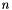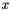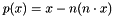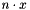ShapeOp  0.1.0
A Tutorial on Adding an Orientation Constraint to ShapeOp

This tutorial will require to rebuild the library. See the ShapeOp Documentation for detailed instructions and requirements Compilation and Installation.

A constraint projection of a given shape is defined as the shape that satisfies the constraint and is closest to the given shape in the least-squares sense. In this tutorial we will implement a orientation constraint. A orientation constraint acts on a set of 3 dimensional point and is satisfied if those points all lie on a plane with a prescribed orientation.

# Mean-centering

Since this constraint only concerns the relative position of points, we can first subtract the mean of all points, project them onto the closest shape satisfying the constraint, and then formulate the global step of ShapeOp with respect to the mean. The global step solves a linear system in the least-square sense. The computation of the mean of a set of given points is also linear, and can therefore be implemented in the linear system.

# Projection

The orientation of a plane can be defined by a normal. After subtracting the mean the least-square fitting plane with normalcontains the origin. The projection of a given pointonto that plane is given by, wheredenotes the dot-product ofand.

# C++ Implementation

All constraints of ShapeOp are implemented in Constraint.h and Constaint.cpp. We therefore add the orientation constraint to those files. In the header file we add the declaration of our class inheriting from the abstract class ShapeOp::Constraint:

class SHAPEOP_API OrientationConstraint : public Constraint {
private:
Vector3 normal_;
mutable Matrix3X input;
};

The private member input is used in the projection function. It ensures that memory is allocated at the construction of the OrientationConstraint object. This has a big impact execution speed, since ShapeOp uses parallelization, while memory allocation is inherently sequential.

The three functions following the constructor are inherited from the parent ShapeOp::Constraint class:

public:
OrientationConstraint(const std::vector<int> &idI,
Scalar weight,
const Matrix3X &positions,
Vector3 normal = Vector3(0.0,0.0,1.0));
virtual ~OrientationConstraint() {}
virtual void project(const Matrix3X & positions, Matrix3X &projections) const override final;
virtual void addConstraint(std::vector<Triplet> &triplets, int &idO) const override final;
void setOrientation(const Vector3 &normal);
};

The last function allows to set a new orientation, which is specific to this constraint. In Constraint.cpp we add the implementation of our OrientationConstraint class. Lets start with the constructor:

SHAPEOP_INLINE OrientationConstraint::OrientationConstraint(const std::vector<int> &idI,
Scalar weight,
const Matrix3X &positions,
Vector3 normal /*= Vector3(0.0,0.0,1.0)*/):
Constraint(idI,weight){
assert(idI.size() >= 1);
setOrientation(normal);
//Allocate memory for intermediate storage during projection
input = Matrix3X::Zero(3, idI.size());
}

The macro SHAPEOP_INLINE allows to inline a function and if applied to all build the whole library inline as a header-only library. The arguments to the constructor are documented in the header file. The setOrientation function is very short:

SHAPEOP_INLINE void OrientationConstraint::setOrientation(const Vector3 &normal){
normal_ = normal;
normal_.normalize();
}

We now add the projection function:

SHAPEOP_INLINE void OrientationConstraint::project(const Matrix3X & positions, Matrix3X &projections) const {
//Copy the constrained positions to input
for (int i = 0; i < static_cast<int>(idI_.size()); ++i) input.col(i) = positions.col(idI_[i]);
//Compute and subtract mean
Vector3 mean_vector = input.rowwise().mean();
input.colwise() -= mean_vector;
//Project each position onto the plane through zero defined by normal_
for (int i = 0; i < static_cast<int>(idI_.size()); ++i){
projections.col(idO_+i) = (input.col(i) - normal_*(normal_.dot(input.col(i))))*weight_;
}
}

ShapeOp uses the Eigen library for linear algebra. Please refer to their documentation for details. Note that the Matrix projections is a global Matrix for all projections in a problem. Each constraint therefore stores an integer id0_ to know where in the global layout of constraints he is. id0_ is stored in the function addConstraint which the solver calls on each constraint so that he adds himself to the linear system used for the global solve in ShapeOp:

SHAPEOP_INLINE void OrientationConstraint::addConstraint(std::vector<Triplet> &triplets, int &idO) const {
//Store this constraints position in the global linear system
idO_ = idO;
//Precompute coefficients for mean-centering
int n_idx = static_cast<int>(idI_.size());
double coef1 = (1.0 - 1.0 / n_idx) * weight_;
double coef2 = -weight_ / n_idx;
//Add triplets to the sparse global linear system.
for (int i = 0; i < n_idx; ++i) {
for (int j = 0; j < n_idx; ++j)
//Add the coefficent for mean-centering to the sparse linear system at column id0 and row idI_[j].
triplets.push_back(Triplet(idO, idI_[j], (i == j ? coef1 : coef2)));
idO++;
}
}

This function sets up part of the global linear system matrix so that if multiplied with the vector of x-coordinates it yields the mean-centered x-coordinate for the corresponding points. The same holds for y- and z-coordinates. The right-hand side of the system is the Matrix projections we saw in the projection constraint. ShapeOp then solves the system - in a least-square sense if it is over-constrained. All code we have added so far yields a working version of our orientation constraint in the C++ part of our ShapeOp library. To support the factory pattern, we add the following line before the return in the static function Constraint::shapeConstraintFactory:

if (constraintType.compare("Orientation") == 0){ if (n < 1) { return c; } return std::make_shared<OrientationConstraint>(idI, weight, positions); }

# C API:

Most interlingual-bindings require C interfaces. ShapeOp has a C interface in the files API.h and API.cpp. Our orientation constraint is already included by the last line of code we added to the factory. To enable editing the orientation normal, we add the following code to the implementation of the function shapeop_editConstraint in API.cpp:

if (strcmp(constraintType, "Orientation") == 0) {
//Trying to cast the constraint given by the id.
auto c = std::dynamic_pointer_cast<ShapeOp::OrientationConstraint>(op->s->getConstraint(constraint_id));
if (!c) { return SO_UNMATCHING_CONSTRAINT_ID; }
//Ensure correct number of scalars provided.
if (nb_scl != 3) { return SO_INVALID_ARGUMENT_LENGTH; }
//Wrap the C-style array of Scalars into a Vector3 and setting the new orientation
Eigen::Map<const ShapeOp::Vector3> n(scalars, 3, 1);
c->setOrientation(n);
return SO_SUCCESS;
}

Now the library needs to be rebuilt. To do so, follow the compilation documentation of ShapeOp Compilation and Installation.

# Grasshopper:

The Grasshopper integration of ShapeOp uses Ironpythons c-types to call our library. First make sure the library loads properly by following the documentation at ShapeOp for Grasshopper(Rhino). Copy the freshly built library to the folder Rhino is loading it from. After loading one of our examples files and running it successfully, the only thing we need to add to use our constraint is an additional option in the Value List component which feeds into the SOCSig Component. To do so, double-click the Value List component and add the following line:

Orientation = "Orientation"

This string is then forwarded without further changes to the SOCSig component, which bundles all parameters for a set of constraints into Constraint-Signatures. The SOSolver component uses the string when adding a new constraint with a call to the dll, where the C API will internally call ShapeOp::Constraint::shapeConstraintFactory where the string ultimately gets resolved.